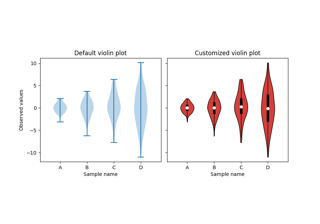# matplotlib.axes.Axes.violinplot¶

Axes.violinplot(self, dataset, positions=None, vert=True, widths=0.5, showmeans=False, showextrema=True, showmedians=False, quantiles=None, points=100, bw_method=None, *, data=None)[source]

Make a violin plot.

Make a violin plot for each column of dataset or each vector in sequence dataset. Each filled area extends to represent the entire data range, with optional lines at the mean, the median, the minimum, the maximum, and user-specified quantiles.

Parameters: datasetArray or a sequence of vectors.The input data. positionsarray-like, default: [1, 2, ..., n]Sets the positions of the violins. The ticks and limits are automatically set to match the positions. vertbool, default: True.If true, creates a vertical violin plot. Otherwise, creates a horizontal violin plot. widthsarray-like, default: 0.5Either a scalar or a vector that sets the maximal width of each violin. The default is 0.5, which uses about half of the available horizontal space. showmeansbool, default: FalseIf True, will toggle rendering of the means. showextremabool, default: TrueIf True, will toggle rendering of the extrema. showmediansbool, default: FalseIf True, will toggle rendering of the medians. quantilesarray-like, default: NoneIf not None, set a list of floats in interval [0, 1] for each violin, which stands for the quantiles that will be rendered for that violin. pointsint, default: 100Defines the number of points to evaluate each of the gaussian kernel density estimations at. bw_methodstr, scalar or callable, optionalThe method used to calculate the estimator bandwidth. This can be 'scott', 'silverman', a scalar constant or a callable. If a scalar, this will be used directly as kde.factor. If a callable, it should take a GaussianKDE instance as its only parameter and return a scalar. If None (default), 'scott' is used. dictA dictionary mapping each component of the violinplot to a list of the corresponding collection instances created. The dictionary has the following keys: bodies: A list of the PolyCollection instances containing the filled area of each violin. cmeans: A LineCollection instance that marks the mean values of each of the violin's distribution. cmins: A LineCollection instance that marks the bottom of each violin's distribution. cmaxes: A LineCollection instance that marks the top of each violin's distribution. cbars: A LineCollection instance that marks the centers of each violin's distribution. cmedians: A LineCollection instance that marks the median values of each of the violin's distribution. cquantiles: A LineCollection instance created to identify the quantile values of each of the violin's distribution.

Notes

Note

In addition to the above described arguments, this function can take a data keyword argument. If such a data argument is given, the following arguments can also be string s, which is interpreted as data[s] (unless this raises an exception): dataset.

Objects passed as data must support item access (data[s]) and membership test (s in data).

## Examples using matplotlib.axes.Axes.violinplot¶